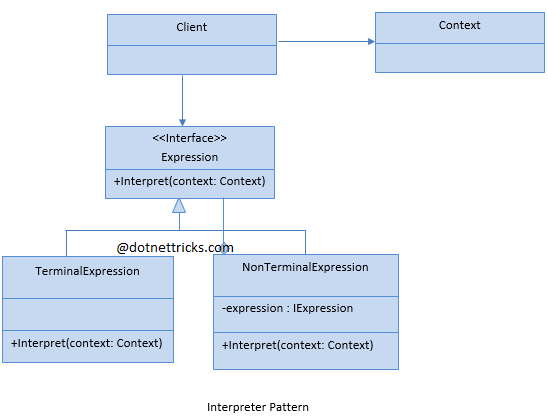# Interpreter

Interpreter Design pattern falls under Behavioral Pattern of Gang of Four (GOF) Design Patterns in .Net. The command pattern is commonly used in the menu systems of many applications such as Editor, IDE, etc. In this article, I would like to share what is interpreter pattern and how is it work?

## What is the Interpreter Design pattern?

Interpreter Design pattern evaluates/ interprets the instructions written in a language grammar or notations. This pattern involves implementing an expression interface which tells to interpret a particular context. This pattern is used in the compilers or parsers or Macro expansions.

This pattern is commonly used in the menu systems of many applications such as Editor, IDE etc.

## Interprets Design Pattern - UML Diagram & Implementation

The UML class diagram for the implementation of the Interprets design pattern is given below:The classes, interfaces, and objects in the above UML class diagram are as follows:

1. ### Client

This is the class that builds the abstract syntax tree for a set of instructions in the given grammar. This tree builds with the help of instances of NonTerminalExpression and TerminalExpression classes.

2. ### Context

This is a class that contains information (input and output), which is used by the Interpreter.

3. ### Expression

This is an interface that defines the Interpret operation, which must be implemented by each subclass.

4. ### NonTerminal

This is a class that implements the Expression. This can have other instances of Expression.

5. ### Terminal

This is a class that implements the Expression.

### C# - Implementation Code

```public class Client
{
public void BuildAndInterpretCommands()
{
Context context = new Context("Dot Net context");
NonterminalExpression root = new NonterminalExpression();
root.Expression1 = new TerminalExpression();
root.Expression2 = new TerminalExpression();
root.Interpret(context);
}
}

public class Context
{
public string Name { get; set; }

public Context(string name)
{
Name = name;
}
}

public interface IExpression
{
void Interpret(Context context);
}

public class TerminalExpression : IExpression
{
public void Interpret(Context context)
{
Console.WriteLine("Terminal for {0}.", context.Name);
}
}

public class NonterminalExpression : IExpression
{
public IExpression Expression1 { get; set; }

public IExpression Expression2 { get; set; }

public void Interpret(Context context)
{
Console.WriteLine("Nonterminal for {0}.", context.Name);
Expression1.Interpret(context);
Expression2.Interpret(context);
}
}

```

## When to use it?

1. Need to interpret a grammar that can be represented as large syntax trees.

2. Parsing tools are available.

3. Efficiency is not a concern.

Related Tutorial
10 Visitor
Contents +